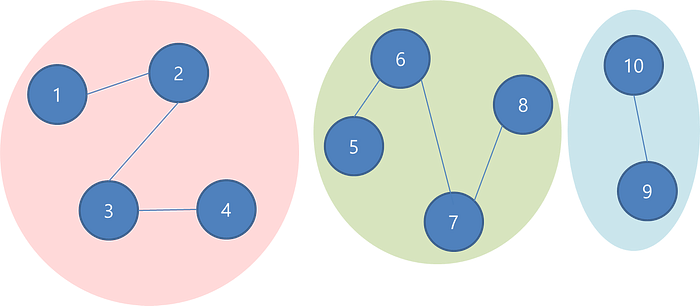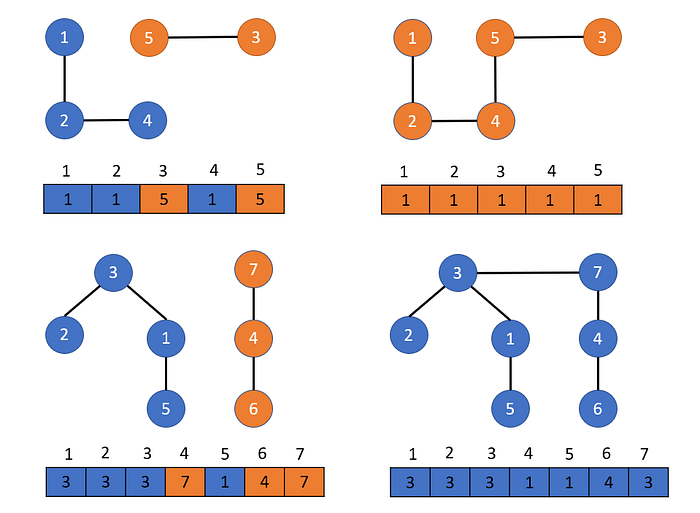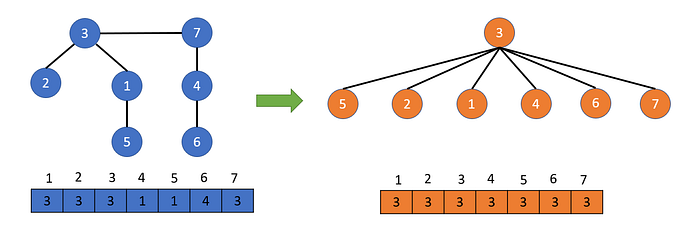# Union Find — Data Structure in PythonA Union-Find/Disjoint set data structure is used to maintain a set of elements partitioned into a number of mutually non-overlapping subsets.

Disjoint Set —A disjoint set is a group of sets where no item can be in more than one set. In other words, if we take any two sets from the Disjoint set then their intersection will result in an empty set. In even simpler terms a disjoint set is a data structure where similar elements are kept in a single group.There are two main operations DSU performs on sets:

Find — Tells us an element/node belongs to which subset.

Union — Join two subsets, if they share a common attribute

In this approach, we represent the nodes using an array, and the value of the item at that index tells which set that node belongs to. Depending upon the strategy used the value may represent its parent or the reference to the representative of the set.### Quick Find

``````class QuickFind():
def __init__(self,N):
self._parents = list(range(0, N))def find(self, p):
return(self._parents[p])

def union(self, p, q):
root_p, root_q = self._parents[p], self._parents[q]
for i in range(0, len(self._parents)):
if(self._parents[i] == root_p):
self._parents[i] = root_q def connected(self,p,q):
return self._parents[p] == self._parents[q]
``````

### Quick Union

``````class QuickUnion():
def __init__(self,N):
self._parents = list(range(0,N))

def find(self, p):
while(p != self._parents[p]):
p = self._parents[p]
return p

def union(self, p, q):
root_p, root_q = self.find(p), self.find(q)
self._parents[i] = root_q def connected(self, p, q):
return self.find(p)==self.find(q)
``````

### Optimized Disjoint Set

We can significantly improve the implementation of the disjoint set by using two techniques:

Union By Rank — Union by rank always attaches the shorter tree to the root of the taller tree.

Path Compression — By making every other node in the path point to its grandparent's node, we can constantly flatten the tree by altering the parent node to the root node and halving the path length.### Weighted Quick Union with optimization

``````class DisJointSets():
def __init__(self,N):
# Initially, all elements are single element subsets
self._parents = [node for node in range(N)]
self._ranks = [1 for _ in range(N)]

def find(self, u):
while u != self._parents[u]:
# path compression technique
self._parents[u] = self._parents[self._parents[u]]
u = self._parents[u]
return u

def connected(self, u, v):
return self.find(u) == self.find(v)

def union(self, u, v):
# Union by rank optimization
root_u, root_v = self.find(u), self.find(v)
if root_u == root_v:
return True
if self._ranks[root_u] > self._ranks[root_v]:
self._parents[root_v] = root_u
elif self._ranks[root_v] > self._ranks[root_u]:
self._parents[root_u] = root_v
else:
self._parents[root_u] = root_v
self._ranks[root_v] += 1
return False
``````

Find — log(N)
Union — log(N)

### Problem 1

N students applied for admission to ABC Academy. An array of integers B is given representing the strengths of N people i.e. B[i] represents the strength of the ith student. Among the N students, some of them knew each other. A matrix C of size M x 2 is given which represents relations where ith relations depict that C[i] and C[i] knew each other. All students who know each other are placed in one batch. The strength of a batch is equal to the sum of the strength of all the students in it. ABC sets criteria for selection: All those batches having a strength of at least D are selected. Find the number of batches selected.

Example:

``````N = 7
B = [1, 6, 7, 2, 9, 4, 5]
C = [[1, 2], [2, 3], [5, 6], [5, 7]]
D = 12Ans: 2
Initial Batches :
Batch 1 = {1, 2, 3} Batch Strength = 1 + 6 + 7 = 14
Batch 2 = {4} Batch Strength = 2
Batch 3 = {5, 6, 7} Batch Strength = 9 + 4 + 5 = 18
Selected Batches are Batch 1 and Batch 2.
``````

We have to create subsets where only students who are friends are in a single subset. Disjoint Sets will be the right choice here.

### Code Implementation

``````def solve(N, B, C, D):
parent = list(range(N))
def find(x):
if x != parent[x]:
parent[x] = find(parent[x])
return parent[x]

def union(x, y):
parent_x = find(x)
parent_y = find(y)
if parent_x != parent_y:
parent[parent_y] = parent_x

for x, y in C:
union(x-1, y-1)

from collections import defaultdict
dict_pair = defaultdict(list)
for idx, val in enumerate(parent):
dict_pair[find(val)].append(B[idx])

res = 0
for v in dict_pair.values():
if sum(v) >= D:
res += 1
return res
``````

Happy Coding!